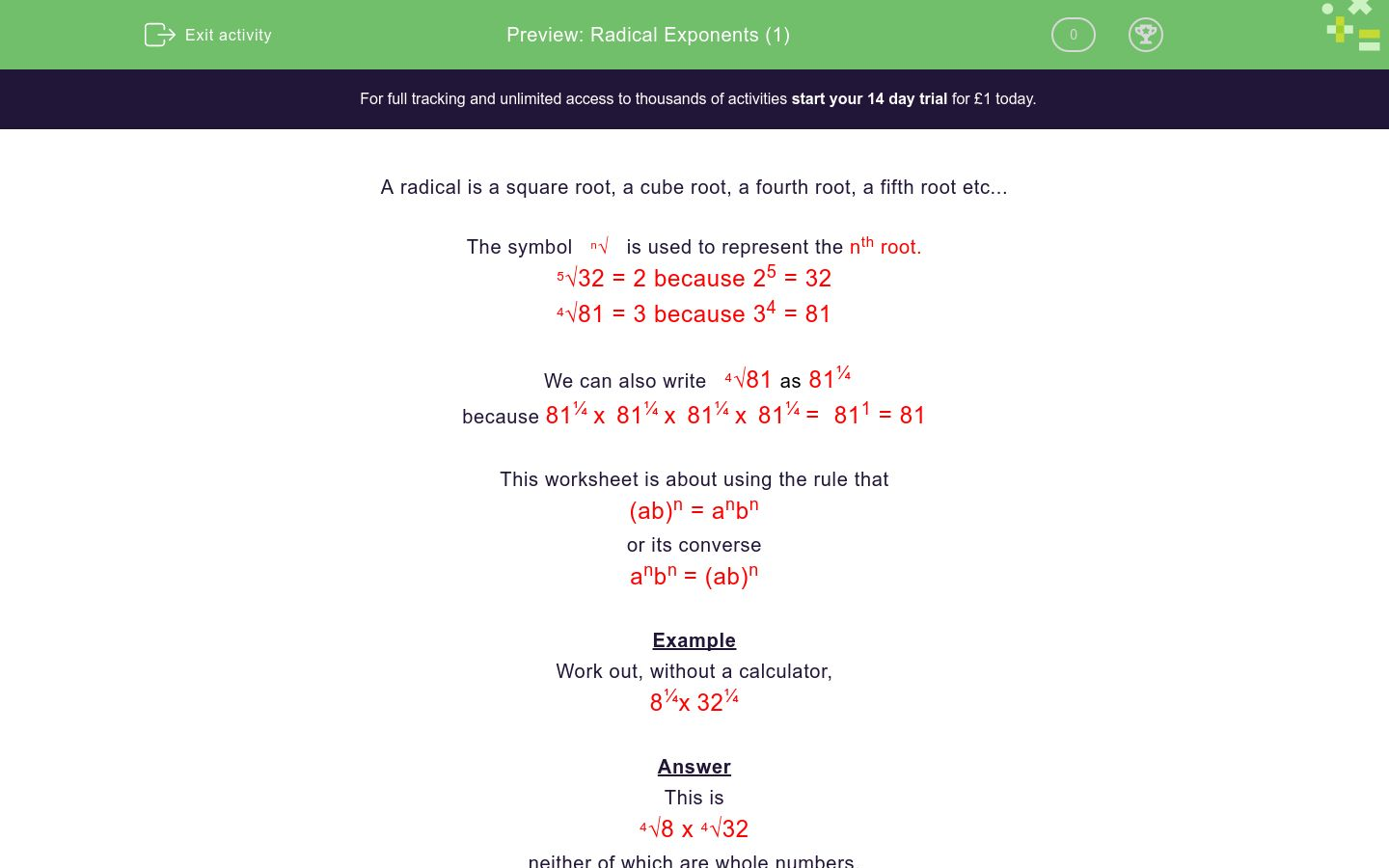### Affordable pricing from £10/month

In this worksheet, students multiply numbers with radical exponents.Key stage:  KS 4

Curriculum topic:  Number

Curriculum subtopic:  Calculate with Roots and Integer (Fractional) Indice

Difficulty level:### QUESTION 1 of 10

A radical is a square root, a cube root, a fourth root, a fifth root etc...

The symbol   n   is used to represent the nth root.

5√32 = 2 because 25 = 32

4√81 = 3 because 34 = 81

We can also write   4√81 as 81¼

because 81¼ x  81¼ x  81¼ x  81¼ =  811 = 81

This worksheet is about using the rule that

(ab)n = anbn

or its converse

anbn = (ab)n

Example

Work out, without a calculator,

8¼x 32¼

This is

4√8 x 4√32

neither of which are whole numbers.

However using the rule above,

8¼x 32¼  =  (8 x 32)¼ =  256¼

4√256 = 4 because 44 = 256

Work out, without a calculator,

8¼ x   2¼

Work out, without a calculator,

1¼ x   1¼

Work out, without a calculator,

3½ x   3½

Work out, without a calculator,

3½ x   12½

Work out, without a calculator,

2½ x   32½

Work out, without a calculator,

2½ x   8½

Work out, without a calculator,

27¼ x   3¼

Work out, without a calculator,

91/3 x   31/3

Work out, without a calculator,

125¼ x   5¼

Work out, without a calculator,

121/3 x   181/3

• Question 1

Work out, without a calculator,

8¼ x   2¼

2
EDDIE SAYS
= (8 × 2)¼
= 16¼
= 4√16
= 2
• Question 2

Work out, without a calculator,

1¼ x   1¼

1
EDDIE SAYS
= (1 × 1)¼
= 1¼
= 4√1
= 1
• Question 3

Work out, without a calculator,

3½ x   3½

3
EDDIE SAYS
= (3 × 3)½
= 9½
= √9
= 3
• Question 4

Work out, without a calculator,

3½ x   12½

6
EDDIE SAYS
= (3 × 12)½
= 36½
= √36
= 6
• Question 5

Work out, without a calculator,

2½ x   32½

8
EDDIE SAYS
= (2 × 32)½
= 64½
= √64
= 8
• Question 6

Work out, without a calculator,

2½ x   8½

4
EDDIE SAYS
= (2 × 8)½
= 16½
= √16
= 4
• Question 7

Work out, without a calculator,

27¼ x   3¼

3
EDDIE SAYS
= (27 × 3)¼
= 81¼
= 4√81
= 3
• Question 8

Work out, without a calculator,

91/3 x   31/3

3
EDDIE SAYS
= (9 × 3)1/3
= 271/3
= 3√27
= 3
• Question 9

Work out, without a calculator,

125¼ x   5¼

5
EDDIE SAYS
= (125 × 5)¼
= 625¼
= 4√625
= 5
• Question 10

Work out, without a calculator,

121/3 x   181/3

6
EDDIE SAYS
= (12 × 18)1/3
= 2161/3
= 3√216
= 6
---- OR ----

Sign up for a £1 trial so you can track and measure your child's progress on this activity.

### What is EdPlace?

We're your National Curriculum aligned online education content provider helping each child succeed in English, maths and science from year 1 to GCSE. With an EdPlace account you’ll be able to track and measure progress, helping each child achieve their best. We build confidence and attainment by personalising each child’s learning at a level that suits them.